# 4. Quick calculations

🥷 In this guide to performing calculations on your data, you'll find explanations for the following functions, as well as examples of how them:

• Row count

• Sum

• Count unique values

• Average

• Max

• Min

To provide context for examples, we'll use the following data table showing reservations for an imaginary car rental company.

## Count rows

The count function counts the total number of rows containing each of the distinct values in a specific column.

For example, you might want to count how many reservations each car make has. To do this, you would click the summarize button, with reservations as the metric and make as the dimension: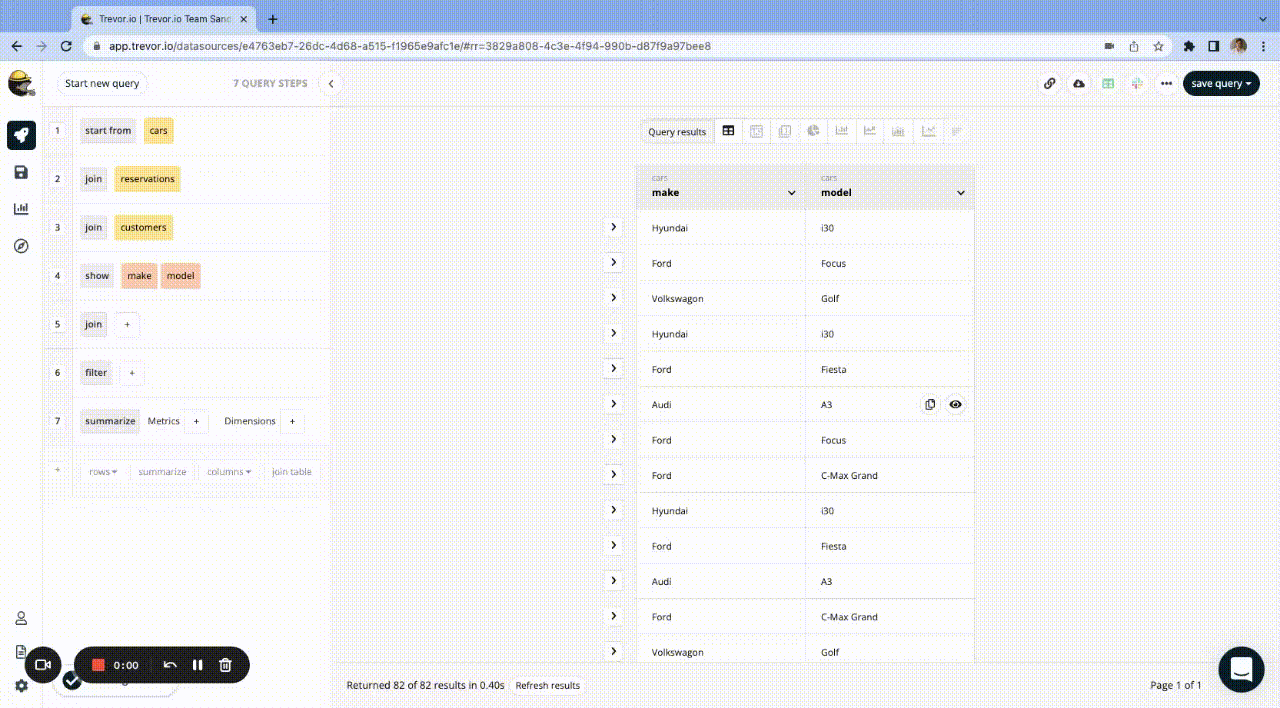To see the number of reservations associated with each of the car brands on each of the pick up days, you would calculate the count broken down by the pick up and also the make column:

## Sum

The sum function sums the values in a specific column.

Examples

To see the total amount paid for reservations for each of the car brands, you would use the summarize button to get the sum of the prices, with the car makes as the dimension: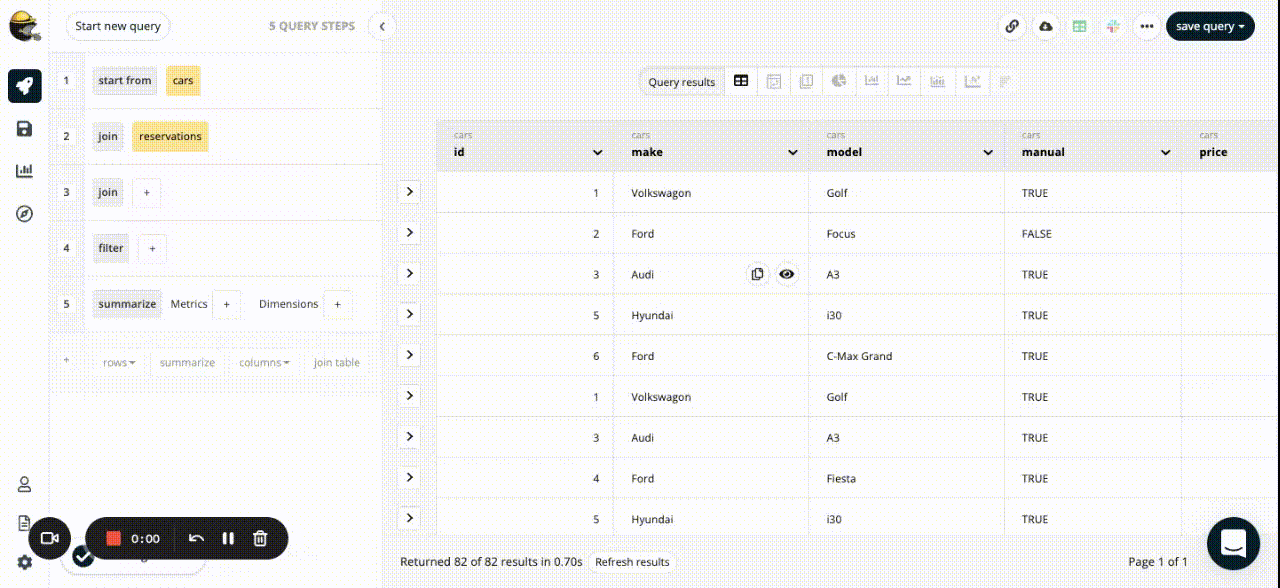## Count unique values

The count unique values function counts the number of unique values in a specific column.

It's particularly useful for situations where a particular value exists in multiple rows but you only want to count it once.

Example

Let's say we wanted to find the amount of revenue each sales person had generated by selling extras (sat nav, child seats etc). To do this, we'd have to join the sales person table, the reservations table, and the extras table together. It would look like this:

To find the total revenue per sales person, we could just calculate the sum of the price column, and break the results down by the name column.

But, what if we wanted to add some extra context by including a count of the reservations? The problem is, we can't simply count the rows by the reservations ID column because, since we've joined the extras table in and each reservation can be associated with multiple extras, it's possible that there are now multiple rows for each reservation. In fact, if you check the first reservation in the table above (ID 81), you'll see it appears in two rows (since it has two extras associated with it).

The solution is to use the count unique values function. So, starting from the table above, we can

1. calculate the sum of the price column, and

2. count the unique values in the reservations ID column, and break both down by the name column.

The result would look like this: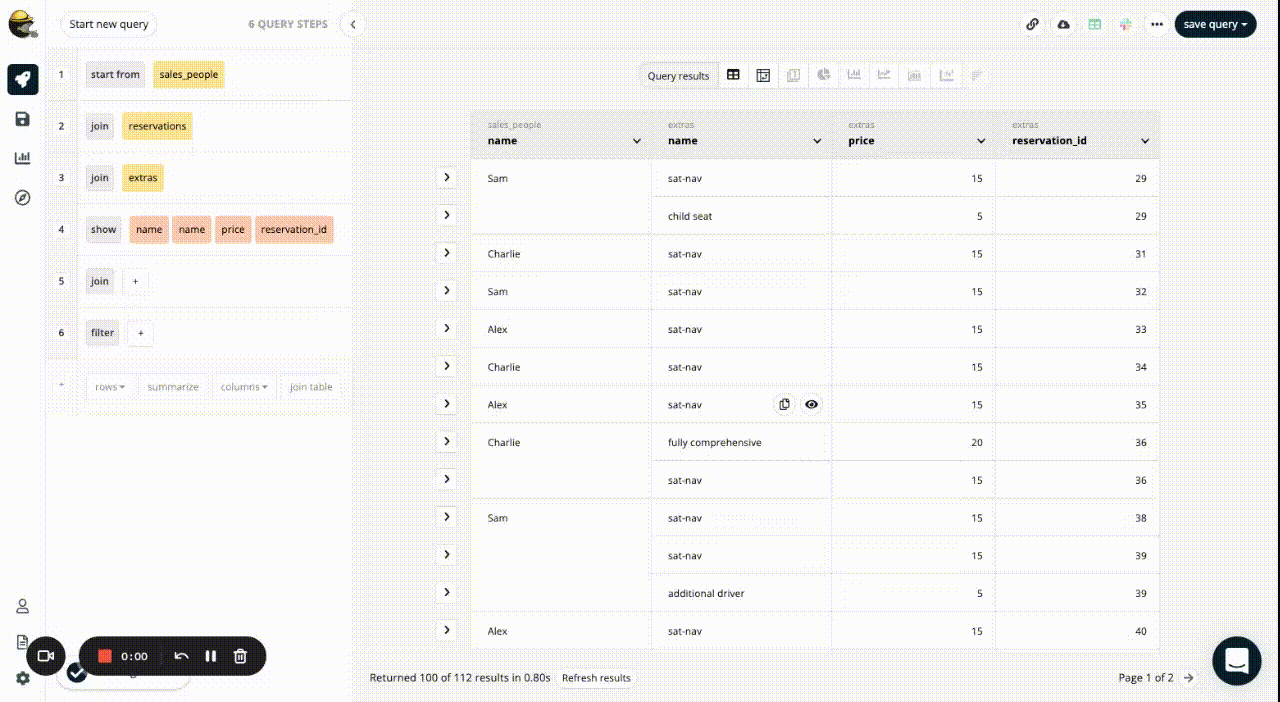## Average

The average function calculates the average of the values in a specific column.

Examples

To see the average price paid per reservation, you would calculate the average of the price column, not broken down by anything: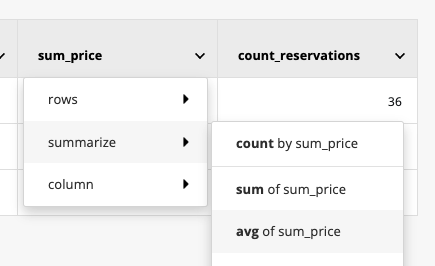To see the average price paid for reservations of each the different car brands, you would calculate the average of the price column broken down by the make column. The result would be:

## Max

The max function returns the highest value in a specific column. If the column contains date values, the highest value is the most recent date.

Example

To see the most recent reservation for each of the car models, you would calculate the max of the pick up column broken down by the model column: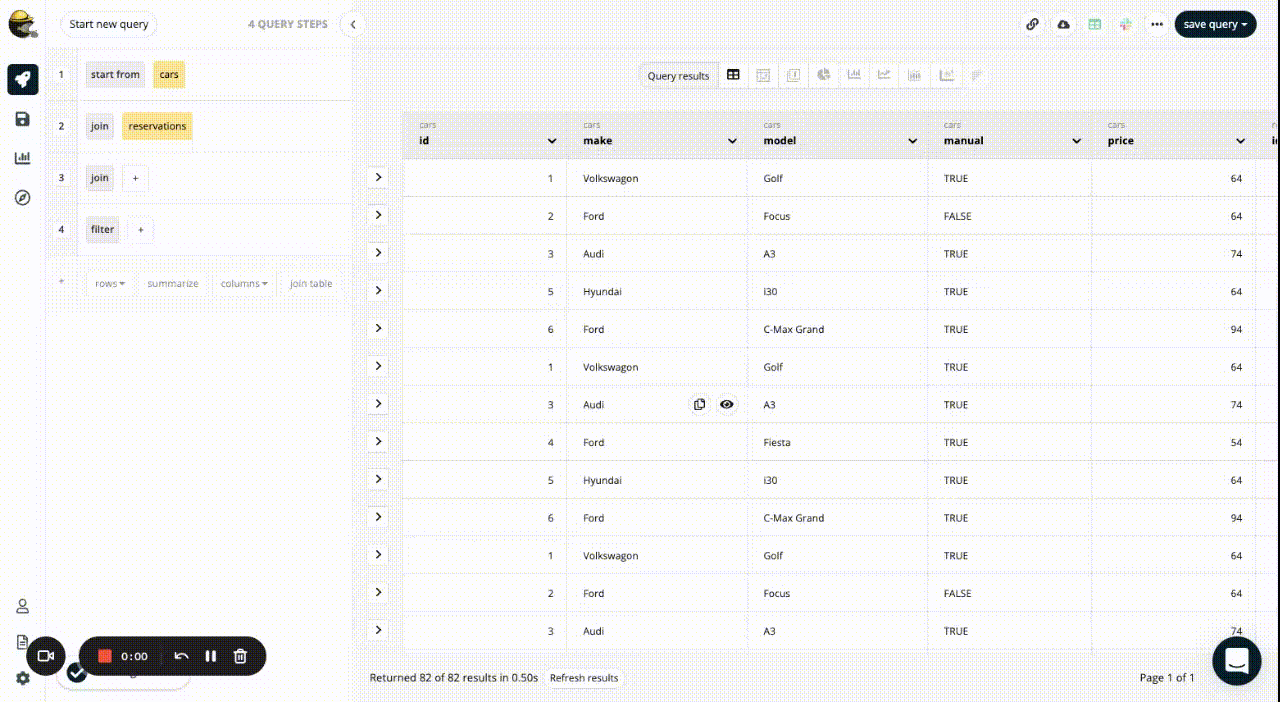## Min

The min function returns the smallest value in a specific column. If the column contains date values, the smallest value is the least recent date.

Examples

To see the lowest price paid on each of the pick up days, you would calculate the min of the price column, broken down by the pick up column: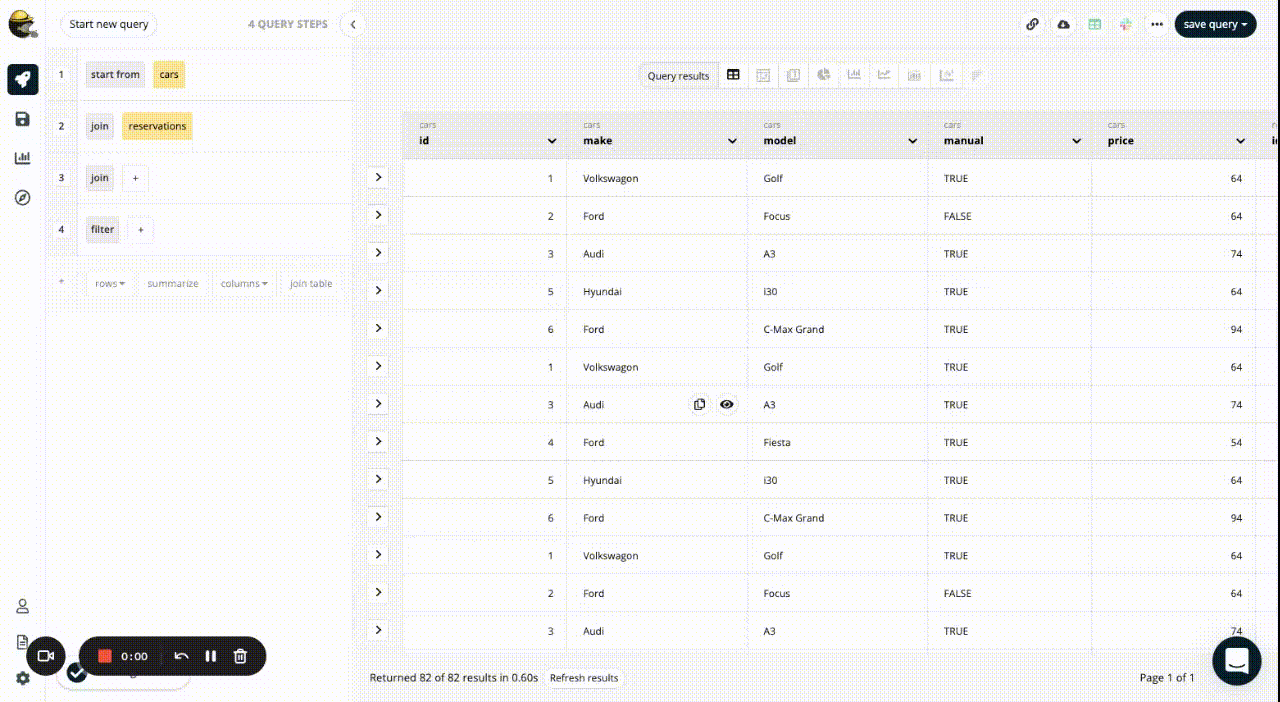0 out of 0 found this helpful

Have more questions? Submit a request

<% if (previousArticle || nextArticle) { %>
<% if (previousArticle) { %>
<% if (previousTitle) { %>

#### <%= previousTitle %>

<% } %> <%= previousArticle.title %>
<% } %> <% if (nextArticle) { %>
<% if (nextTitle) { %>

#### <%= nextTitle %>

<% } %> <%= nextArticle.title %>
<% } %>
<% } %>
<% if (items.length > 1) { %>